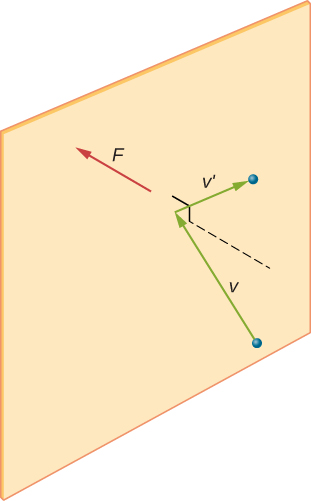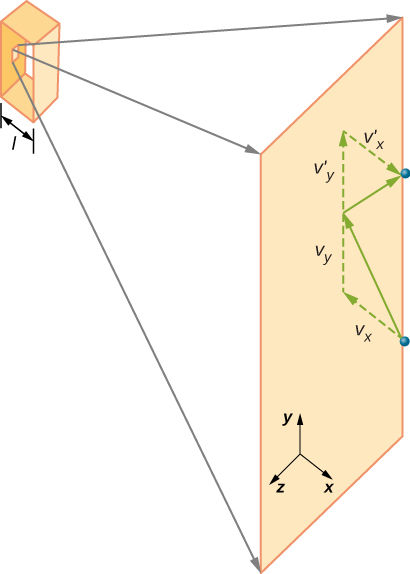# 2.2 Pressure, temperature, and rms speed  (Page 2/18)

 Page 2 / 18When a molecule collides with a rigid wall, the component of its momentum perpendicular to the wall is reversed. A force is thus exerted on the wall, creating pressure.

In a sample of gas in a container, the randomness of the molecular motion causes the number of collisions of molecules with any part of the wall in a given time to fluctuate. However, because a huge number of molecules collide with the wall in a short time, the number of collisions on the scales of time and space we measure fluctuates by only a tiny, usually unobservable fraction from the average. We can compare this situation to that of a casino, where the outcomes of the bets are random and the casino’s takings fluctuate by the minute and the hour. However, over long times such as a year, the casino’s takings are very close to the averages expected from the odds. A tank of gas has enormously more molecules than a casino has bettors in a year, and the molecules make enormously more collisions in a second than a casino has bets.

A calculation of the average force exerted by molecules on the walls of the box leads us to the ideal gas law and to the connection between temperature and molecular kinetic energy. (In fact, we will take two averages: one over time to get the average force exerted by one molecule with a given velocity, and then another average over molecules with different velocities.) This approach was developed by Daniel Bernoulli (1700–1782), who is best known in physics for his work on fluid flow (hydrodynamics). Remarkably, Bernoulli did this work before Dalton established the view of matter as consisting of atoms.

[link] shows a container full of gas and an expanded view of an elastic collision of a gas molecule with a wall of the container, broken down into components. We have assumed that a molecule is small compared with the separation of molecules in the gas, and that its interaction with other molecules can be ignored. Under these conditions, the ideal gas law is experimentally valid. Because we have also assumed the wall is rigid and the particles are points, the collision is elastic (by conservation of energy—there’s nowhere for a particle’s kinetic energy to go). Therefore, the molecule’s kinetic energy remains constant, and hence, its speed and the magnitude of its momentum remain constant as well. This assumption is not always valid, but the results in the rest of this module are also obtained in models that let the molecules exchange energy and momentum with the wall.Gas in a box exerts an outward pressure on its walls. A molecule colliding with a rigid wall has its velocity and momentum in the x -direction reversed. This direction is perpendicular to the wall. The components of its velocity momentum in the y - and z -directions are not changed, which means there is no force parallel to the wall.

If the molecule’s velocity changes in the x -direction, its momentum changes from $\text{−}m{v}_{x}$ to $+m{v}_{x}.$ Thus, its change in momentum is $\text{Δ}mv=+m{v}_{x}-\left(\text{−}m{v}_{x}\right)=2m{v}_{x}.$ According to the impulse-momentum theorem given in the chapter on linear momentum and collisions, the force exerted on the i th molecule, where i labels the molecules from 1 to N , is given by

what is principle of superposition
what are questions that are likely to come out during exam
what is electricity
watt is electricity.
electricity ka full definition with formula
Jyoti
If a point charge is released from rest in a uniform electric field will it follow a field line? Will it do so if the electric field is not uniform?
Maxwell's stress tensor is
Yes
doris
neither vector nor scalar
Anil
if 6.0×10^13 electrons are placed on a metal sphere of charge 9.0micro Coulombs, what is the net charge on the sphere
18.51micro Coulombs
ASHOK
Is it possible to find the magnetic field of a circular loop at the centre by using ampere's law?
Is it possible to find the magnetic field of a circular loop at it's centre?
yes
Brother
The density of a gas of relative molecular mass 28 at a certain temperature is 0.90 K kgmcube.The root mean square speed of the gas molecules at that temperature is 602ms.Assuming that the rate of diffusion of a gas in inversely proportional to the square root of its density,calculate the density of
A hot liquid at 80degree Celsius is added to 600g of the same liquid originally at 10 degree Celsius. when the mixture reaches 30 degree Celsius, what will be the total mass of the liquid?
Under which topic
doris
what is electrostatics
Study of charges which are at rest
himanshu
Explain Kinematics
Two equal positive charges are repelling each other. The force on the charge on the left is 3.0 Newtons. Using your notes on Coulomb's law, and the forces acting on each of the charges, what is the force on the charge on the right?
Using the same two positive charges, the left positive charge is increased so that its charge is 4 times LARGER than the charge on the right. Using your notes on Coulomb's law and changes to the charge, once the charge is increased, what is the new force of repulsion between the two positive charges?
Nya
A mass 'm' is attached to a spring oscillates every 5 second. If the mass is increased by a 5 kg, the period increases by 3 second. Find its initial mass 'm'
a hot water tank containing 50,000g of water is heated by an electric immersion heater rated at 3kilowatt,240volt, calculate the current
what is charge
product of current and time
JaffarBy OpenStaxByBy OpenStaxBy OpenStaxBy OpenStaxBy OpenStaxBy David BourgeoisBy Caitlyn GobbleBy John GabrieliBy